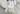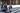# How AI can power your customer experience

By Christopher Lacy-Hulbert

Earlier this year, Zenitech founder and CTO Christopher Lacy-Hulbert shared how we took a new look into product search.

‘What if search was driven by lifestyle and went deeper than just matching a bunch of words against an index?’ — we asked ourselves.

As a result, we built AutoCompare — an application that helps you find the most suitable vehicle to fit your lifestyle by prioritising criteria.

This is a follow-up piece, shedding a little more light on how we powered the engine of product search and discovery based on lifestyle preferences rather than on product qualities.

## Purpose of the algorithm

AutoCompare can be used for two main purposes:

1. Make sense of raw data in order to assign a vehicle to a category.
2. Find vehicles that would suit users’ preferences the best.

## Raw data processing

This is the first step in our process, as we need to make sure we have some data prepared before we can search it and give some suggestions for users. What we aim to achieve in this step is to find a way to make sense of overwhelming amount of characteristics that are used to describe a vehicle and give us some kind of prediction for how well this car would suit every category we have listed in lifestyle search. For example, we would probably expect a fancy Lamborghini to be classified as being very sporty and neither eco nor family friendly. This prediction process should be quick enough, as the market is flooded with tens of thousands of different vehicles all of which should be evaluated for every lifestyle category, we have in AutoCompare. We do not want to hard-code rules that would check a couple of predefined fields such as engine capacity or 0–60 time and tell if that car is sporty or not.

• First of all, this requires some technical knowledge to determine if one of many characteristics in raw data makes vehicle more of lifestyle category X or maybe a bit more towards lifestyle category Y (e.g. would you think that vehicle that has 1st gear ratio 4.38 to 1 is more of a supercar or something really eco-friendly? No clue? I have no idea as well).
• This hard-coded rule approach also makes us throw away all the data we are not using in our predefined category-deciding rules. This is a problem as there might be valuable information about lifestyle category in some of the parameters we failed to identify as important for the category.
• And the final drawback is that this approach is completely non reusable — if we decide to build similar tool for choosing a mobile phone, or checking real estate market or any other field that has objects described by a large set of parameters, we basically need to start from scratch and adapt hard-coded rules for the new market.

## Neural Networks

An obvious solution for the above problems is Neural networks. Using Neural networks, we no longer must analyze raw data by ourselves, but rather pass this responsibility to a Neural network.

However, there is still one more step before we can start classifying all the vehicles in our database $\Bbb{D}$ — we have to supply the network with some training data. Since our goal is to get an evaluation for every category in the lifestyle categories list, the network is expected to approximate a function:

$F(v)=c$

where v is a vector of parameters, representing a vehicle we are trying to categorize:

$v=(v_1, v_2,, v_n).$

$c=(c_1, c_2, ..., c_m), c_i \in \R : c_i \in [0,1], i \in [1, 2, ..., m]$ Given this array of characteristics, the output should be lifestyle category vector $c$:

Every value $c_i$ represents how well vehicle $v$ suits category $i$. Let’s say we know that first category represents family-friendliness. Then if we get an array like [0.99, 0.5, 0.3, …] which has a number close to 1 in first position — we understand that the network thinks this vehicle should perform really well in family-friendly category. On the other hand — having a value close to 0 in some position i would mean this vehicle being bad at $i$-th category.

Before running this function F on every car record $v \in \Bbb{D}$ we must make sure the Neural network has at least a rough idea of what is a good example of a vehicle that is very suitable for every category.

## Training

First step in building a Neural network approximator is picking a set of vehicles:

$\Bbb{V} = v_1, v_2,, v_k |v_1 \in \Bbb{D}$

to manually classify.

Having a set of vehicles V we can build a training data set

$\Bbb{X}=x_1, x_2,, x_k$

where

$x_i = (c_1, c_2, ..., c_m), c_i \in [0,1], m$ number of categories.

$x_i \in \Bbb{X}$ represents a category vector that we classified as ground truth for vehicle $v_i$. Once we have a number of different vehicles labeled in the training set $\Bbb{X}$ we can pass this set to the Neural network to learn — adjust its inner set of weights $\theta$ so that output

$F_\theta(v)$
$\lim_{t\to\infin} F_\theta(v)=x_v$ would be as close as possible to $x_v$:

where t is number of Neural network learning iterations performed.

During this process, the Network considers every field in a vehicle datasheet and works out how important it is for a certain category cᵢ.

Once we are happy with how the Network categorizes vehicles in the dataset, we can easily get category numbers for every entry in our database:

vehicles = D.getAllEntries();
c = vehicles.map(v => F(v));


And here we arrive at the second problem AutoCompare solves — performing lifestyle search according to user’s preferences.

What we end up with after categorizing every vehicle in database is a set of vectors:

$\Bbb{C} = c_1, c_2,, c_v, |\Bbb{C}|=|\Bbb{V}|, \text{where } c_i = (c_1, c_2,, c_m)$

here $c_i$ represents a single vehicle $v_i \in \Bbb{V}$

## Searching multidimensional space for closest matches

Here is where user’s lifestyle choices array comes into play. Once a user has provided his preferences for lifestyle categories, AutoCompare converts that preference list into a vector l, using function $\Bbb{G}$:

$l =G(choices) = (l_1, ..., l_m), l_i \in \Reals$

which, just like vehicle category vectors, is m-dimensional vector:

$dim(l) = dim(c) = m, \forall c \in \Complex$

It also has the highest value in the position representing category that user identified as the most important, the second highest number for category that user listed second in search, etc.:

$l_i \ge l_j \iff ord(i) < ord(j)$

where $l = (l_1, l_2, ..., l_m)$,

$ord(C) = n, n\in \Z$

— function that returns in which position user placed category C during lifestyle search.

User’s lifestyle preference vector l is the search goal — AutoCompare should suggest vehicles $V^* = { v_1, v_2, ..., v_n }$ that have category vector $F(v) = c$ as close as possible to this goal:

$V^* = \{ v_1, v_2, ..., v_n ||| l-F(v) || < || l-F(v') ||, \forall v' \in \Bbb{V} \backslash V^*, \forall v \in V^* \}$

Brute-force method for this search would be calculating the Euclidean distance between our goal l and every vehicle vector c and searching for points that have the smallest Euclidean distance from our goal. However, this approach does not scale at all.

An approach we’ve chosen is storing our vehicle vectors in a data structure called k-dimensional which makes nearest neighbour search for multidimensional space really easy.

## Closing note

It was a fascinating journey to get from raw, seemingly unrelated data sets to advanced Neural networks and search multidimensional space. We are happy we took the hard way there though. As in the end we have a mechanism that is scalable and can be applied in different domains.